Courses

# Behaviour of Real Gases Deviation from Ideal Gas Class 11 Notes | EduRev

## Class 11 : Behaviour of Real Gases Deviation from Ideal Gas Class 11 Notes | EduRev

The document Behaviour of Real Gases Deviation from Ideal Gas Class 11 Notes | EduRev is a part of the Class 11 Course Chemistry for JEE.
All you need of Class 11 at this link: Class 11

6. Deviations from Ideal Behaviour

An ideal gas is one which obeys the gas laws of the gas equation PV = RT at all pressure and temperatures. However, no gas in nature is ideal. Almost all gases show significant deviations from the ideal behaviour. Thus the gases H2, N2 and CO2 which fail to obey the ideal-gas equation are termed as non-ideal or real gases.

Compressibility Factor : The extent to which a real gas departs from the ideal behaviour may be depicted in terms of a new function called the compressibility factor, denoted by Z. It is defined as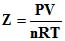The deviations from ideality may be shown by a plot of the compressibility factor Z, against P.

For an ideal gas, Z = 1 and it is independent of temperature and pressure.

The deviations from ideal behaviour of a real gas will be determined by the value of Z being greater or less than 1.

The difference between unity and the value of the compressibility factor of a gas is a measure of the degree of non-ideality of the gas.

For a real gas, the deviations from ideal behaviour depends on :

(i) pressure; and (ii) temperature.

This will be illustrated by examining the compressibility curves of some gases discussed below with the variation of pressure and temperature.

Effect of Pressure Variation on Deviations: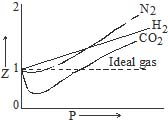Effect of Temperature on Deviations: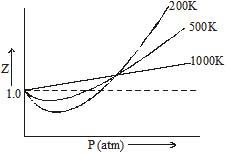From the above curves we can conclude that:

1. At low pressure and fairly high temperatures, real gases show nearly ideal behaviour and the ideal-gas equation is obeyed.

2. At low temperatures and sufficiently high pressures, a real gas deviates significantly from ideality and the ideal-gas equation is no longer valid.

3. The closer the gas is to the liquefaction point, the larger will be the deviation from the ideal behaviour.

Greater is the departure of Z from unity, more is the deviation from ideal behaviour.

(i) When Z < 1, this implies that gas is more compressible.

(ii) When Z > 1, this means that gas is less compressible.

(iii) When Z = 1, the gas is ideal.

Vander Waals Equation of State for a Real Gas: The equation of state generated by Vander Waals in 1873 reproduces the observed behaviour with moderate accuracy. For n moles of gas, the Vander Waals equation is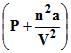(V - nb) = nRT

where and b are constants characteristic of a gas. This equation can be derived by considering a real gas and converting it to an ideal gas.

Volume Correction : We know that for an ideal gas P x V = nRT. Now in a real gas the molecular volume cannot be ignored and therefore let us assume that `b' is the volume excluded (out of the volume of container) for the moving gas molecules per mole of a gas. Therefore due to n moles of a gas the volume excluded would be nb.

∴ a real gas in a container of volume V has only available volume of (V - nb) and this can be thought of, as an ideal gas in a container of volume (V - nb)

Pressure Correction: Let us assume that the real gas exerts a pressure P. The molecules that exert the force on the container will get attracted by molecules of the immediate layer which are not assumed to be exerting pressure.

It can be seen that pressure the real gas exerts would be less than the pressure an ideal gas would have exerted. Therefore if a real gas exerts a pressure P, then an ideal gas would exert a pressure equal to P p(p is the pressure lost by the gas molecules due to attractions). This small pressure p would be directly proportional to the extent of attraction between the molecules which are hitting the container wall and the molecules which are attracting these.

Therefore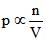(concentration of molecules which are hitting the container's wall)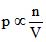(concentration of molecules which are attracting these molecules )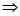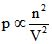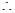where a is the constant of proportionality which depends on the nature of gas. Higher value of `a' reflects the increased attraction between gas molecules.

The Vander Waals constant b (the excluded volume) is actually 4 times the volume of a single molecule. i.e. b = 4 NAwhere NA xx Avogadro number.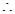b = 4 x 6.023 x 1023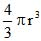, where  r  is the radius of a molecule.

The constant a and b: Vander Waals constant for attraction (A) and volume (B) are characteristic for a given gas. Some salient features of `a' and `b' are:

(i) For a given gas Vander Waal's constant of attraction `a' is always greater than Vander Waals constant of volume (B).

(ii) The gas having higher value of `a' can be liquified easily and therefore H2 and He are not liquified easily.

(iii) The units of a = litre2 atm mole-2 and that of b = litre mole-1

(iv) The numerical values of a and b are in the order of 10-1 to 10-2 to 10-4 respectively.

(v) Higher is the value of `a' for a given gas, easier is the liquefaction.

Explanation of deviation by Van der Waals equation

(i) At lower pressure : `V' is large and `b' is negligible in comparison with V.

Then Vander Waals equation reduces to :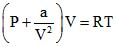⇒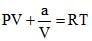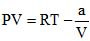⇒ z =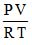= 1 -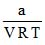or PV < RT  at low pressure (below Boyle temperature)

this accounts for the dip in PV  vs  P isotherm at low pressure.

(ii)  At fairly high pressures :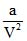may be neglected in comparison with P.

The Vander Waals equation becomes

P ( V-b) = RT ⇒ PV - Pb = RT

PV = RT Pb ⇒ z == 1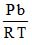or PV > RT at higher pressure (above Boyle temperature)

This accounts for the rising parts of the PV vs P isotherm at high pressures.

(iii) At very low pressure :  V becomes so large that both b and become negligible and the Vander Waals equation reduces to PV = RT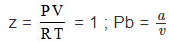At extremely low pressure (at Boyle temperature)

This shows why gases approach ideal behaviour at very low pressures.

(iv) Hydrogen and Helium : These are two lightest gases known. Their molecules have very small masses. The attractive forces between such molecules will be extensively small. Sois negligible even at ordinary temperatures. Thus PV > RT.

Dieterici Equation :

P (V - nb) = n R T ea/VRT (for `n' mole of a gas)

Berthelot Equation :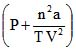(V - nb) = n R T

Virial Equation Of State For 1 Mole Of Gas :

z == 1 B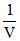C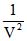D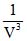..............

B = second virial co-efficient , temperature dependent = b -

C = third virial co - efficient, temperature dependent = b2

Illustration 16 : The compressibility factor for 1 mole of a van der Waals gas at 0xC and 100 atm pressure is found to be 0.5. Assuming that the volume of a gas molecule is negligible, calculate the van der Waals constant, a.

Solution: For 1 mole of the gas,

Z =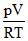⇒ 0.5 =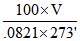; V = 0.112 L

Neglecting  b, van der Waals equation reduces to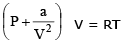or pV  +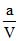= RT

or 100 x 0.112 +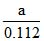= .0821 x 273

a = 1.25 L2atm mol-2

Offer running on EduRev: Apply code STAYHOME200 to get INR 200 off on our premium plan EduRev Infinity!

## Chemistry for JEE

223 videos|452 docs|334 tests

,

,

,

,

,

,

,

,

,

,

,

,

,

,

,

,

,

,

,

,

,

;